# 从零开始学习 zk-SNARK（一）——多项式的性质与证明

## 导读

17 年最早接触 zk-SNARK 开始，就断断续续得学习了一些 zk-SNARK 的知识，但对其原理始终存在诸多困惑，没有形成一个完整的认识。偶然一次机会，看到了 Maksym Petkus 的这篇文章。文章从最基本的多项式性质讲起，从一个简单易懂的证明协议开始，然后像堆积木一样在发现问题，修改问题中逐步去完善协议，直到最终构造出完整的 zk-SNARK 协议。另外作者这种从问题出发的讲解方式，让读者知其然，也知其所以然 。作为一枚毕业多年早把数学知识还给老师的程序媛，读到这篇文章如获至宝，这篇文章读下来的感受像找到了一个脚手架，将脑海里碎片化的知识逐渐拼凑完整。于是想把它翻译出来（已获得作者授权），一方面加深自己的学习，另一方面也将这份宝藏分享给小伙伴们。

—— even@安比实验室

## Maksym（作者）：

From Extractable Collision Resistance to Succinct Non-Interactive Arguments of Knowledge, and Back Again ;
Pinocchio: Nearly Practical Verifiable Computation

zkSNARKs in a nutshell ;
Quadratic Arithmetic Programs: from Zero to Hero ;
Zk-SNARKs: Under the Hood ;
Explaining SNARKs Part I: Homomorphic Hidings

## 序言和介绍

Zero-knowledge succinct non-interactive arguments of knowledge (zk-SNARK) 确实是一种非常精妙的方法，它可以在不揭示任何信息的前提下证明某个论断为真。但首要问题是，它为什么有用？

1. 证明关于隐私数据的声明：

• 一个人 A 的银行账户金额多于 X
• 去年，一家银行未与实体 Y 进行交易
• 在不暴露全部 DNA 数据的前提下匹配 DNA
• 一个人的信用评分高于 Z
2. 匿名认证：

• 在不揭露身份的情况下（比如登录密码），证明请求者 R 有权访问网站的受限区域
• 证明一个人来自一组被允许的国家/地区列表中的某个国家/地区，但不暴露具体是哪个
• 证明一个人持有地铁月票，而不透露卡号
3. 匿名支付：

• 付款完全脱离任何一种身份
• 纳税而不透露收入
4. 外包计算

• 将昂贵的计算外包，并在不重新执行的情况下验证结果是否正确；它打开了一种零信任计算的类别
• 改进区块链模型，从所有节点做同样的计算，到只需一方计算然后其它节点进行验证

• 完整性 —— 只要「陈述」是正确的，prover 就可以让 verifier 确信
• 可靠性 —— 如果「陈述」是错误的，那么作弊的 prover 就没有办法让 verifier 相信
• 零知识 —— 协议的交互仅仅揭露「陈述」是否正确而不泄漏任何其它的信息

## 证明的媒介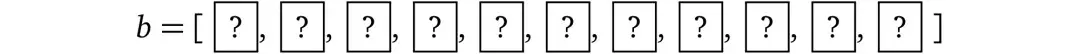verifier 一次只能检查（即，读）一位。为了验证这个陈述，我们以某种任意的顺序读取元素并检查其是否确实等于 1 。如果第一次抽样检查的结果是 1，就设置「陈述」的可信度为 ⅒= 10％，否则，如果等于 0，就说明「陈述」是错误的。验证者继续进行下一轮验证，直到获得足够的可信度为止。假如在一些场景下要信任 prover 需要至少 50% 的可信度，那就意味着必须执行 5 次校验。但假如在其它一些场景下需要 95% 的可信度，就需要检查所有的元素。很明显这个证明协议的缺点是必须要根据元素的数量进行检查，如果我们处理数百万个元素的数组，这么做是不现实的。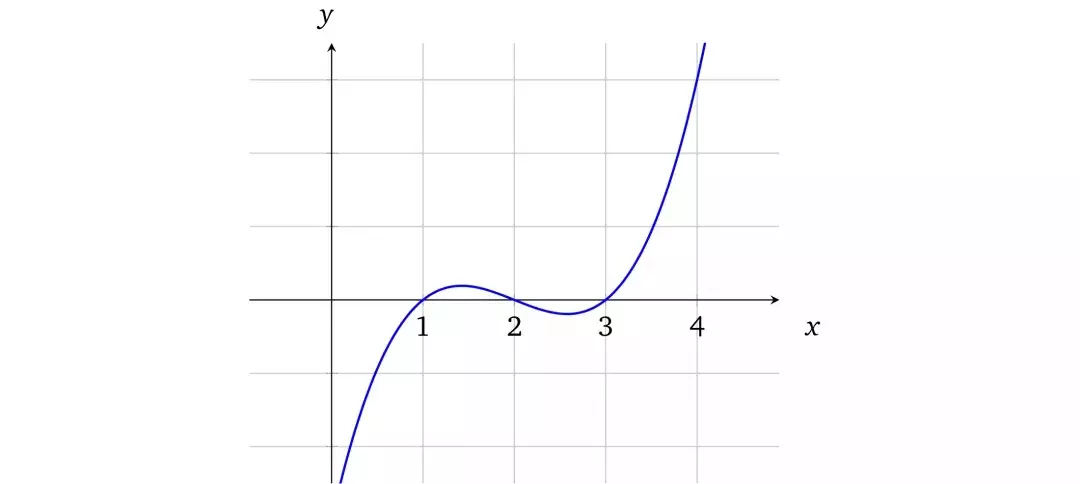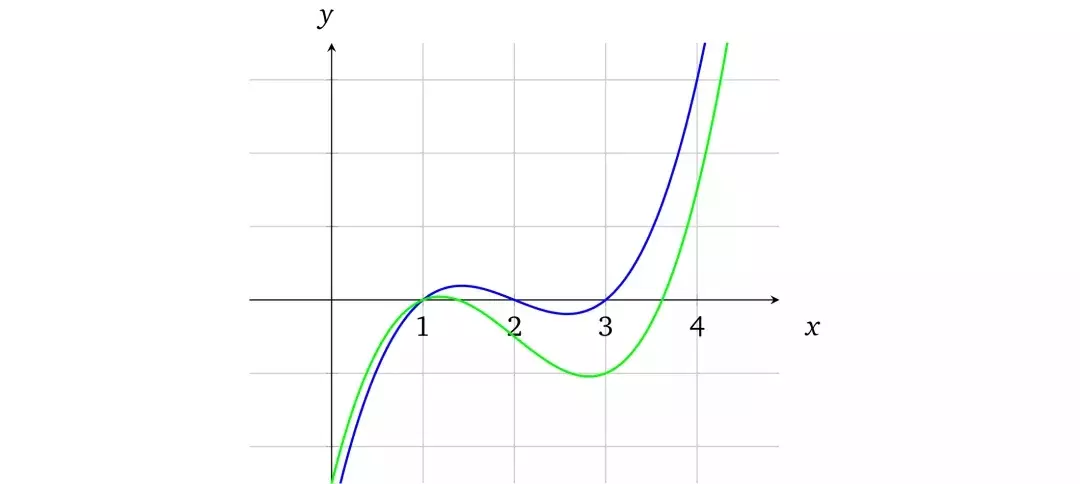x³ – 6x² + 11x – 6 =x³ – 6x² + 10x – 5
x-1=0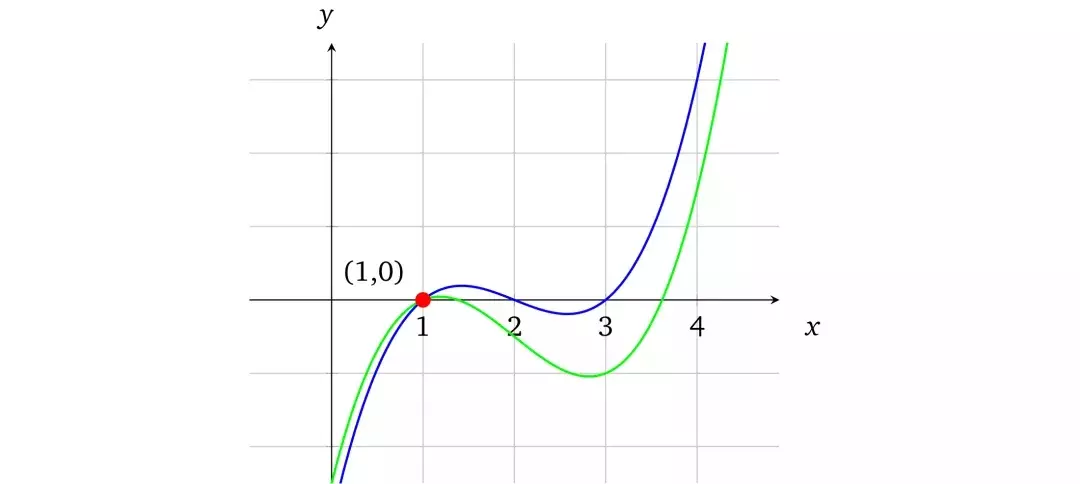x³ – 6x² +11x - 6 = 504
x³ – 6x² +10x - 5 = 495

• verifier 选择一个随机值 x 并在本地计算多项式结果
• verifier 将 x 值给到 prover，并让他计算相关的多项式结果
• prover 代入 x 到多项式计算并将结果给到 verifier
• verifier 检查本地的计算结果和 prover 的计算结果是否相等，如果相等那就说明 prover 的陈述具有较高的可信度

@Maksym（作者）

even@安比实验室：这一节告诉了我们多项式的一个重要性质：我们不可能找到共享连续段的两条不相等曲线，也就是任何多项式在任意点的计算结果都可以看做是其唯一身份的表示。也就是说只要能证明多项式上的某个随机点就可以证明这个多项式（只有在知道了多项式，才能算出这个点对于的值），这个性质是我们下面所有证明的核心。

## 证明多项式的知识

$$c_nx^n+ .. +c_1x^1+c_0x^0$$

even@安比实验室：这一节告诉了我们多项式的一个本质——多项式的「知识」就是多项式的系数。所谓「知道」多项式就是指「知道」多项式的系数。

## 因式分解

$$(x-a_0)(x-a_1) ..(x-a_n)=0$$

$$x^3 - 3x^2 + 2x =(x-0)(x-1)(x-2)$$

(x-1)(x-2) · …

p(x) = t(x) · h(x)

$$h(x)=\frac{p(x)}{t(x)}$$

even@安比实验室：多项式可以被因式分解成它的根的因式的乘积。这个性质就意味着，如果一个多项式有某些解，那么它被因式分解后的式子中一定包含这些解的因式。

• verifier 挑选一个随机值 r, 计算 t = t(r) (即，求值) ，然后将 r 发送给 prover。
• prover 计算 h(x) =p(x) / t(x) ，并对 p(r) 和 h(r) 进行求值，将计算结果 p, h 提供给 verifier。
• verifier 验证 p= t⋅h，如果多项式相等，就意味着 t(x) 是 p(x) 的因式。

• verifier 选一个随机数 23，并计算 t = t(23) = (23 – 1)(23 – 2) = 462，然后将 23 发给 prover
• prover 计算 h(x) =p(x) / t(x) = x, 并对 p(r) 和 h(r) 进行求值，p= p(23) = 10626，h= h(23) = 23，将 p 和 h 提供给 verifier
• verifier 再验证 p= t⋅h：10626 = 462 ⋅ 23 是正确的，这样陈述就被证明了。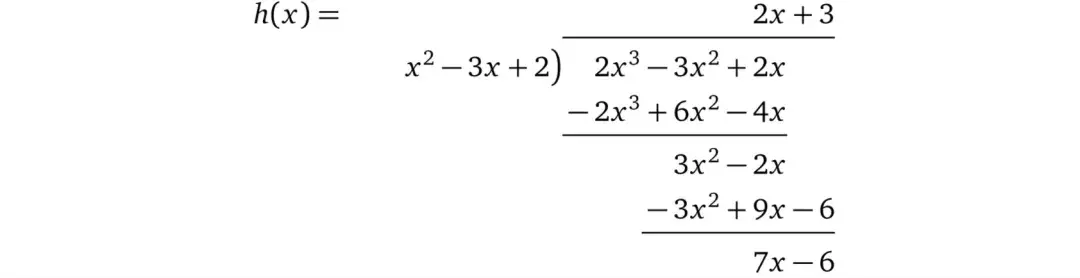@Maksym（作者）

$$h(x)=2x+3+\frac{7x-6}{t(x)}$$ 。

Remark 3.1现在我们就可以在不知道多项式的前提下根据特定的性质来验证多项式了，这就已经给了我们一些零知识和简明性的特性。但是，这个结构中还存在好多问题：

• prover 可能并不知道他所声称的 p(x)，他可以先算一下 t = t(r)，然后选择一个随机值 h，由此计算出 p = t⋅h。因为等式是成立的，所以也能通过 verifier 的校验。
• 因为 prover 知道随机点 x = r ，他可以构造出一个任意的多项式，这个任意多项式与 t(r) ⋅ h(r) 在 r 处有共同点。
• 在前面的「陈述」中，prover 声称他知道一个特定阶数的多项式，但现在的协议对阶数并没有明确的要求。因而 prover 完全可以拿一个满足因式校验的更高阶数的多项式来欺骗 verifier。

even@安比实验室：利用因式的性质构造出了一个证明协议，但这个协议存在一些缺陷，主要是由于

1. prover 知道了 t(r)，他就可以反过来任意构造一个可以整除 t(r) 的 p(r)
2. prover 知道了点(r,t(r) · h(r)) 的值，就可以构造经过这一点的任意多项式，同样满足校验
3. 协议并没有对 prover 的多项式阶数进行约束

## 模糊计算

Remark 3.1 中的前两个问题是由于 暴露了原始值 而导致的，也就是 prover 知道了 r 和 t(r)。但如果 verifier 给出的这个值像放在黑盒里一样不可见的话就完美了，也就是一个人即使不破坏协议，也依然能在这些模糊的值上面完成计算。有点类似哈希函数，从计算结果就很难再回到原始值上。

### 同态加密

5³=125

$$125^2 = 15625 = {(5^3)}^2 =5^{2\times3} = 5^6$$

$$5^3\cdot 5^2 = 5^3+2 = 5^5 = 3125$$

$$\frac{5^5}{5^3} = 5^5\cdot5^{-3} = 5^{5-3} = 5^2 =25$$

### 模运算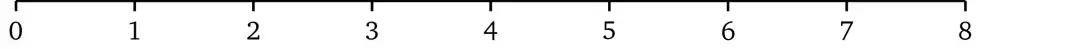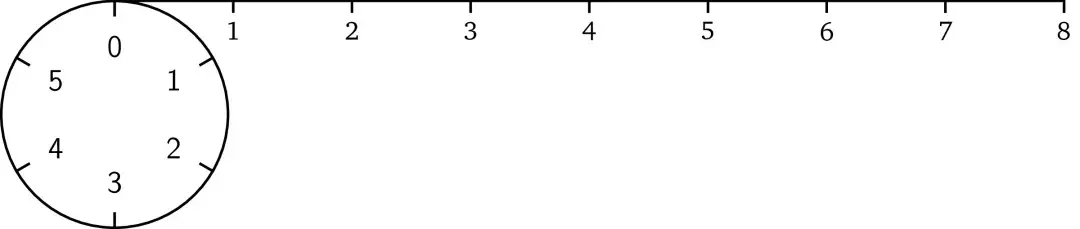3 × 5 = 3 mod 6
5 + 2 = 1 mod 6

2 × 4 = 1 mod 6
2 - 1 = 1 mod 6
1 × 3 = 3 mod 6

5 × 4 = 2 mod 6
4 × 2 = 2 mod 6
2 × 1 = 1 mod 6
……

### 强同态加密

$$5^1 = 5(mod 7)$$
$$5^2 = 4(mod 7)$$
$$5^3 = 6(mod 7)$$
……

$$5^5 = 3(mod 7)$$
$$5^{11} = 3(mod 7)$$
$$5^{17} = 3(mod 7)$$
……

encryption: $$5^3 = 6 (mod 7)$$
multiplication: $$6^2 = (5^3)^2 = 5^6 = 1 (mod 7)$$
addition: $$5^3\cdot5^2=5^5=3(mod7)$$

$$E(v) = g^v (mod n)$$

Remark 3.2 这个同态加密模式有一个限制，我们可以把一个加密的值和一个未加密的值相乘，但我们不能将两个加密的值相乘（或者相除），也就是说我们不能对加密值取幂。虽然这些性质第一感觉看起来很不友好，但是这却构成了 zk-SNARK 的基础。这个限制后面将在“加密值乘法”一节中讲到。

even@安比实验室：通过模运算形成的集合被称为「有限域」，而通过计算指数再进行模运算形成的集合构成「循环群」。常见的同态加密方式除了整数幂取模之外，还有椭圆曲线上的倍乘。

### 加密多项式

$$E {(x^3)}^1\cdot E {(x^2)}^{-3} \cdot E {(x)}^2 = {({g^x}^3)}^1 \cdot {({g^x}^2)}^{-3} \cdot {(g^x)}^2 = g^{1x^3} \cdot (g^{-3x^2}) \cdot {g^x}^2 = g^{x^3-3x^2+2x}$$

• Verifier

• 取一个随机数 s ，也就是秘密值

• 指数 i 取值为 0，1，…，d 时分别计算出 s 的 i 次幂的加密结果，即：$$E{(s^i)}=g^i$$

• 代入 s 计算未加密的目标多项式：$$t(s)$$

• 将对 s 的幂的加密值提供给 prover:$$E{(s^0)},E{(s^1)},..,E{(s^d)}$$

• Prover

• 计算多项式 h(x) = p(x)/t(x)

• 使用加密值$${g^s}^0,{g^s}^1,..,{g^s}^d,$$和系数 $$c_0,c_1,..,c_n$$计算

• 然后同样计算$$E(h(s)) =g^h(s)$$

• 将结果 $$g^p$$ 和 $$g^h$$ 提供给 verifier

• Verifier

• 最后一步是 verifier 去校验

$$p=t(s) \cdot h:g^p ={(g^h)}^{t(s)}=>g^p=g^{t(s)\cdot h}$$

even@安比实验室：利用强同态加密这个工具，构造了一个相对较强的零知识证明协议。但是如上文所述，这里还是存在一些问题—— 无法验证 prover 是否是真的使用了 verifier 提供的值来构造证明的。

https://arxiv.org/pdf/1906.07221.pdf
why-and-how-zk-snark-works-1-introduction
why-and-how-zk-snark-works-2-proving-knowledge-of-a-polynomial

【译】完全理解以太坊智能合约 1年以前  |  735次阅读

Substrate 入门（5）- 区块头 1年以前  |  703次阅读